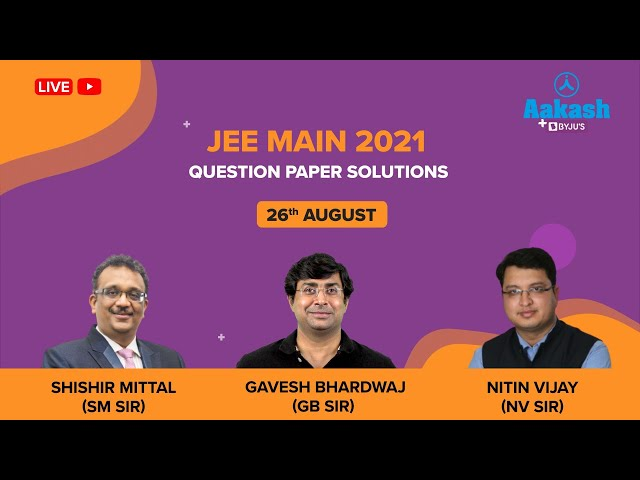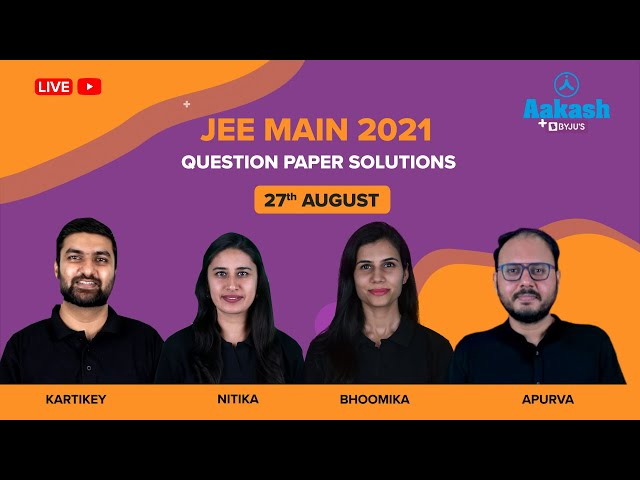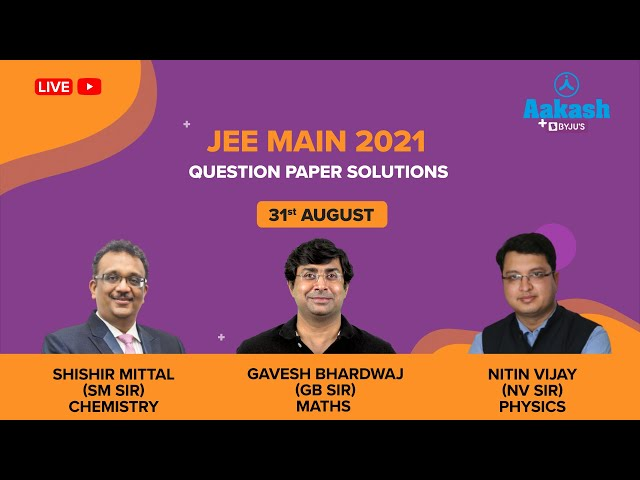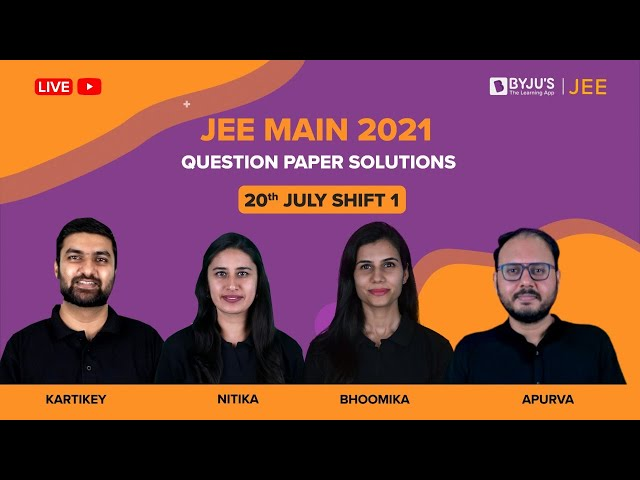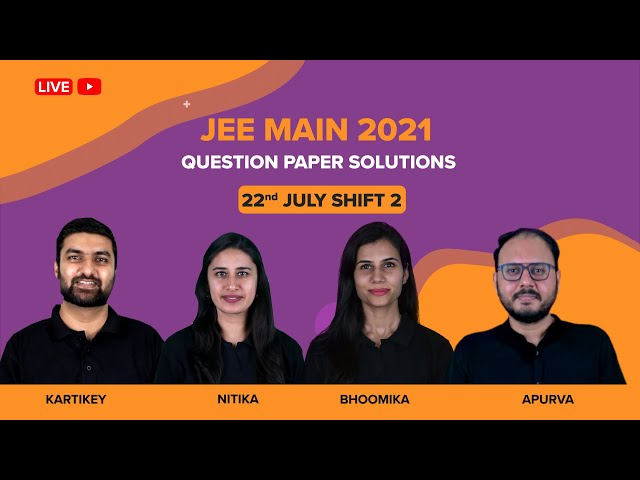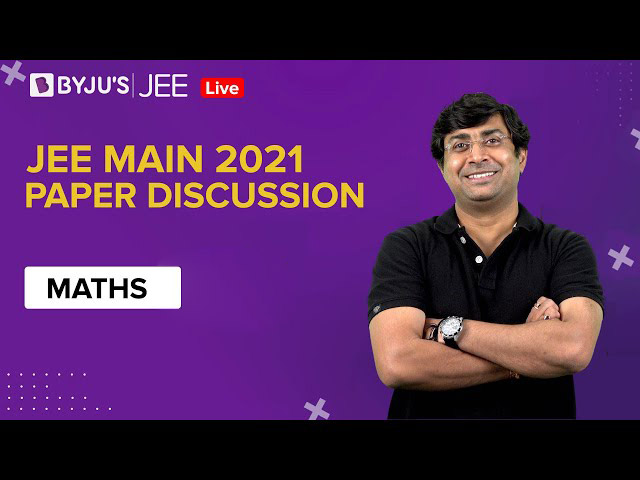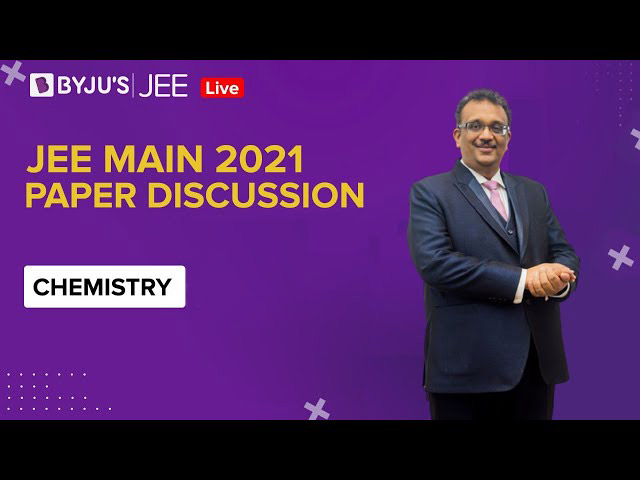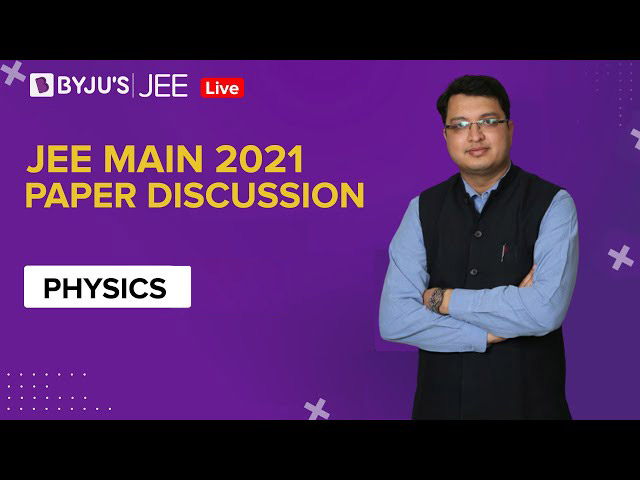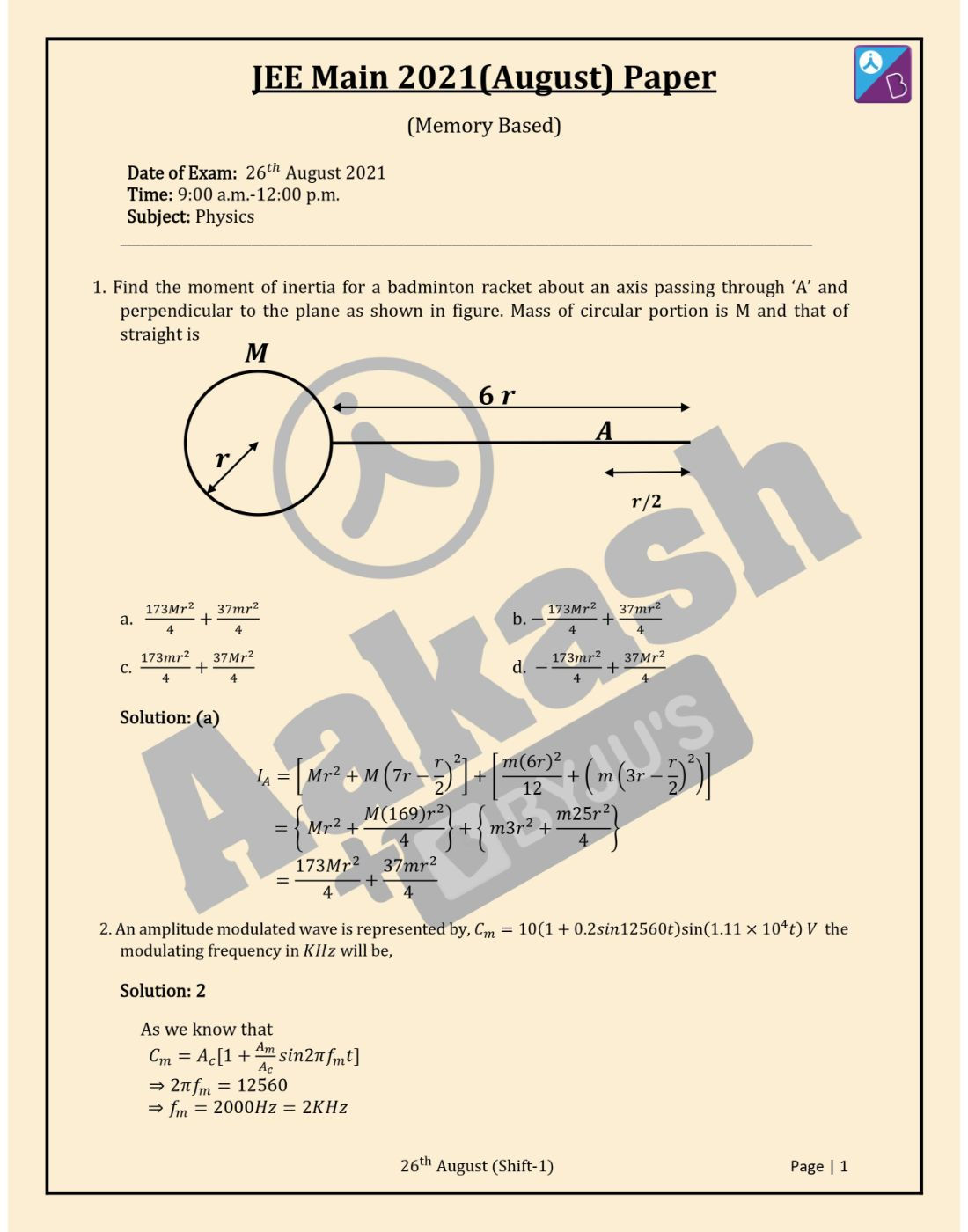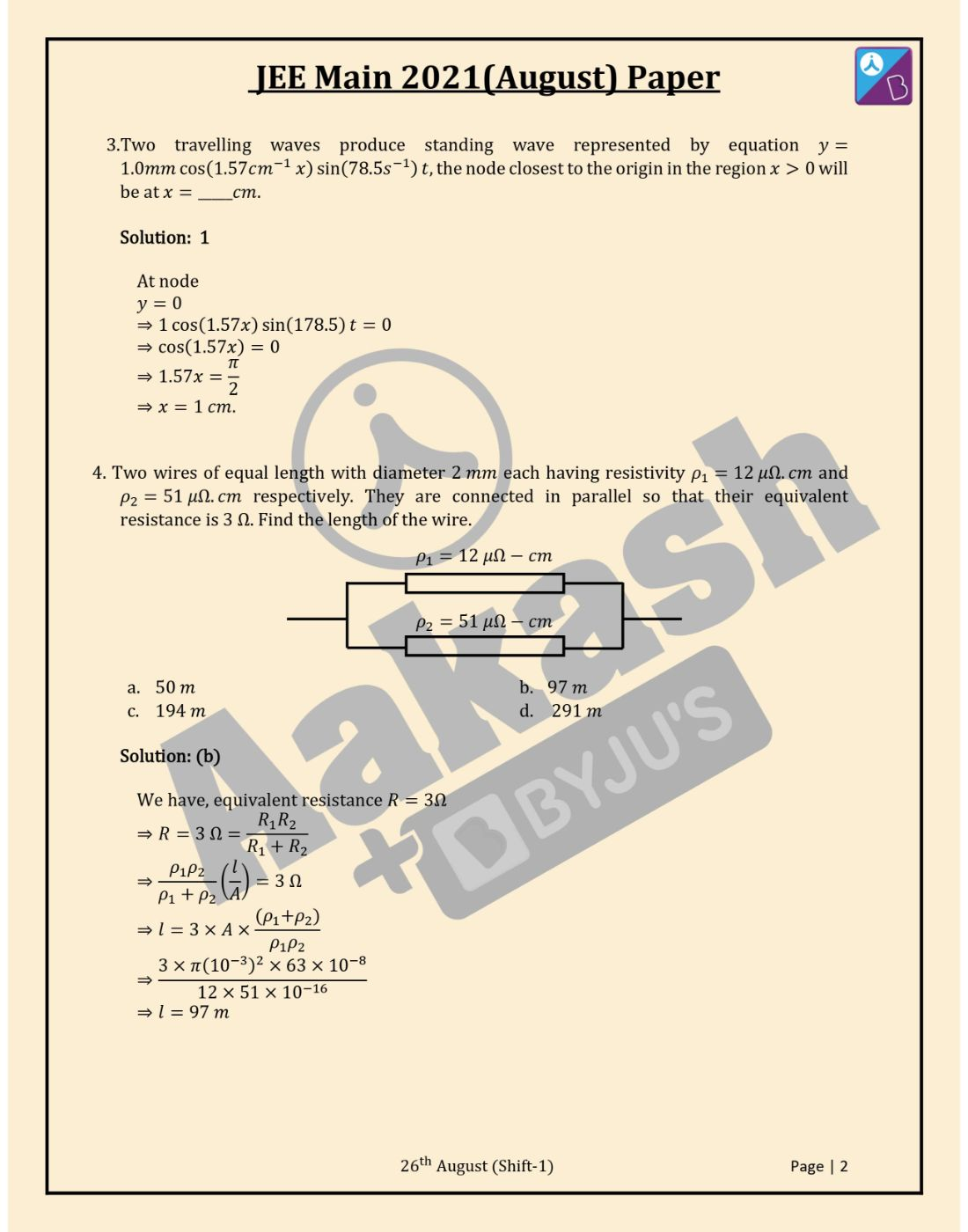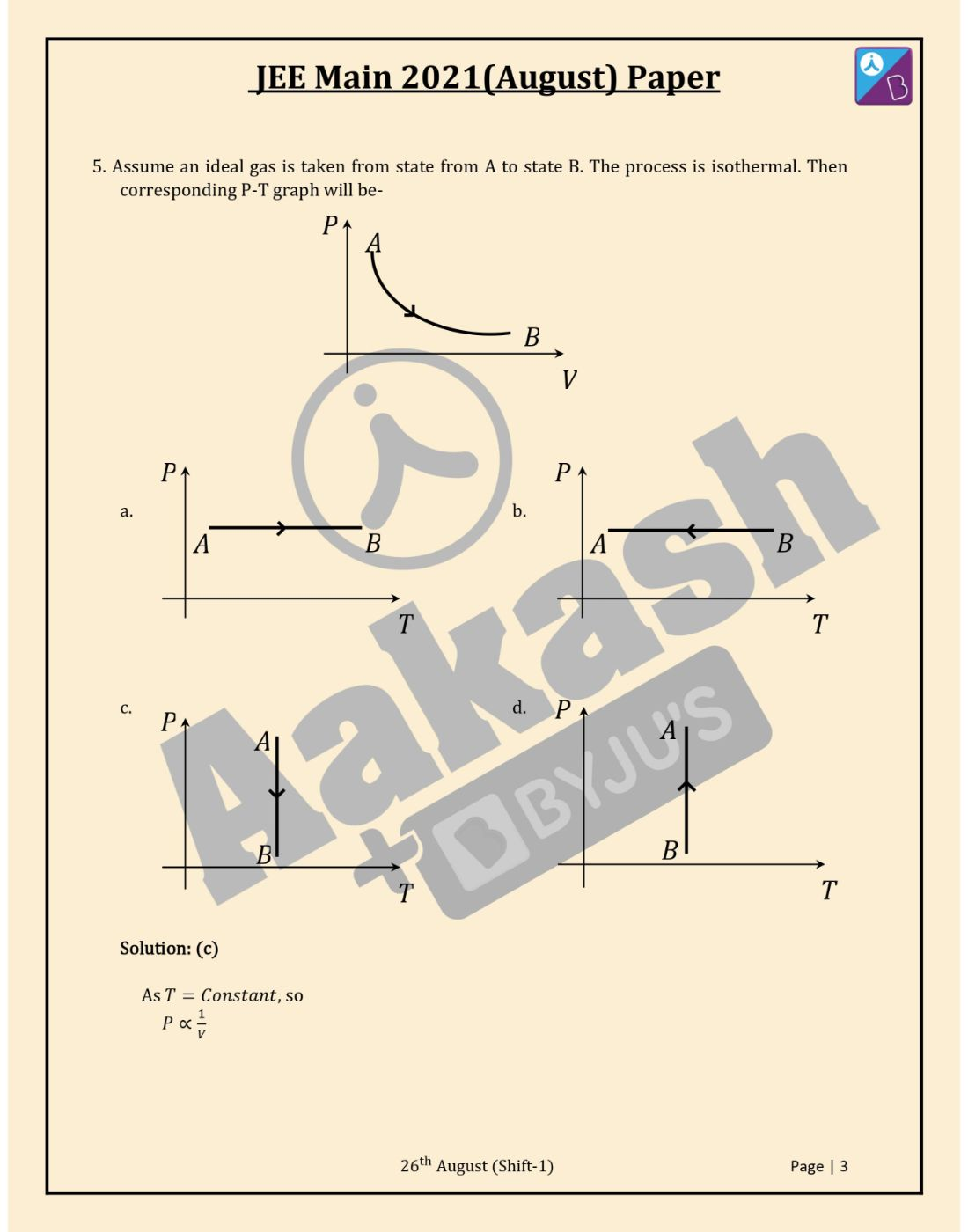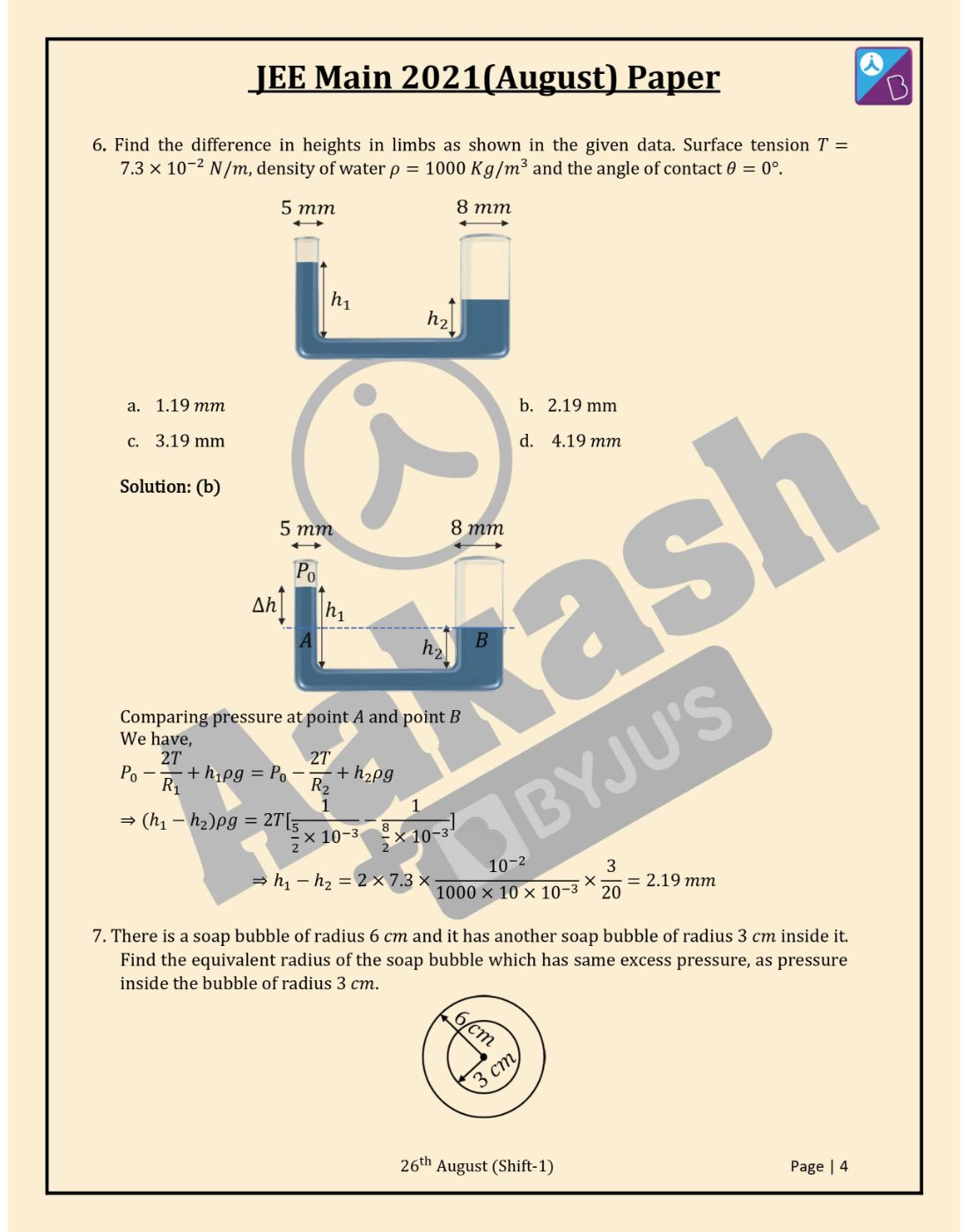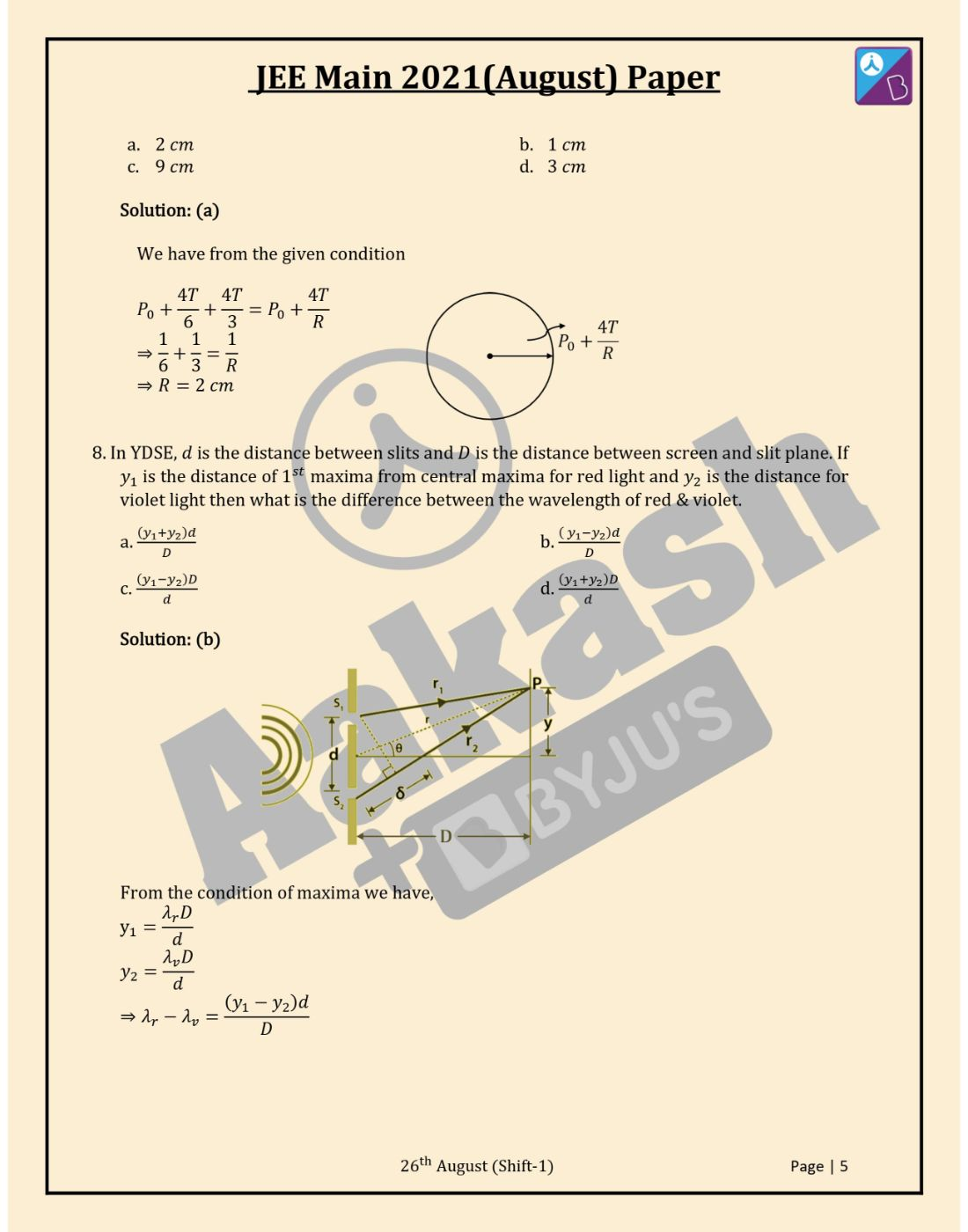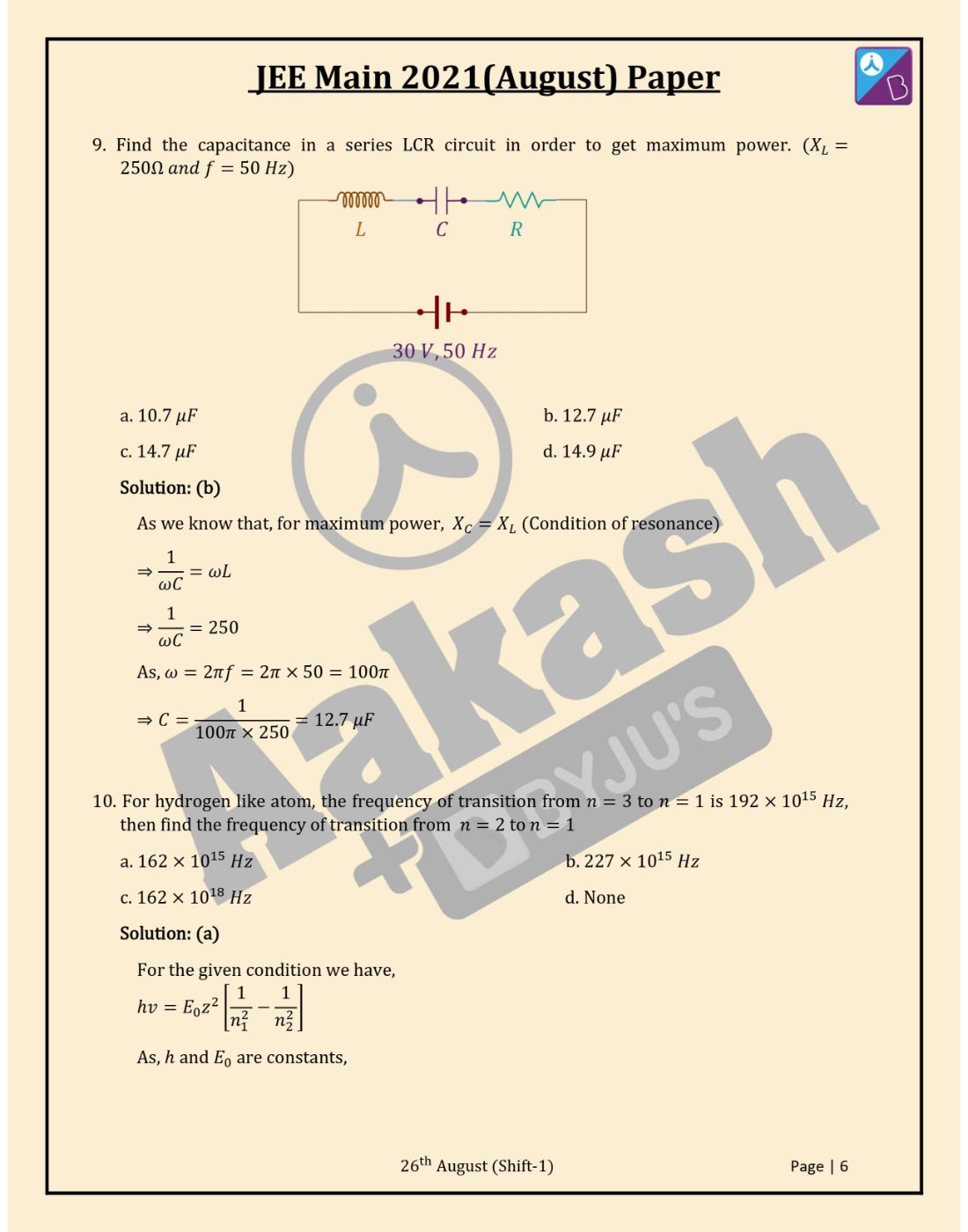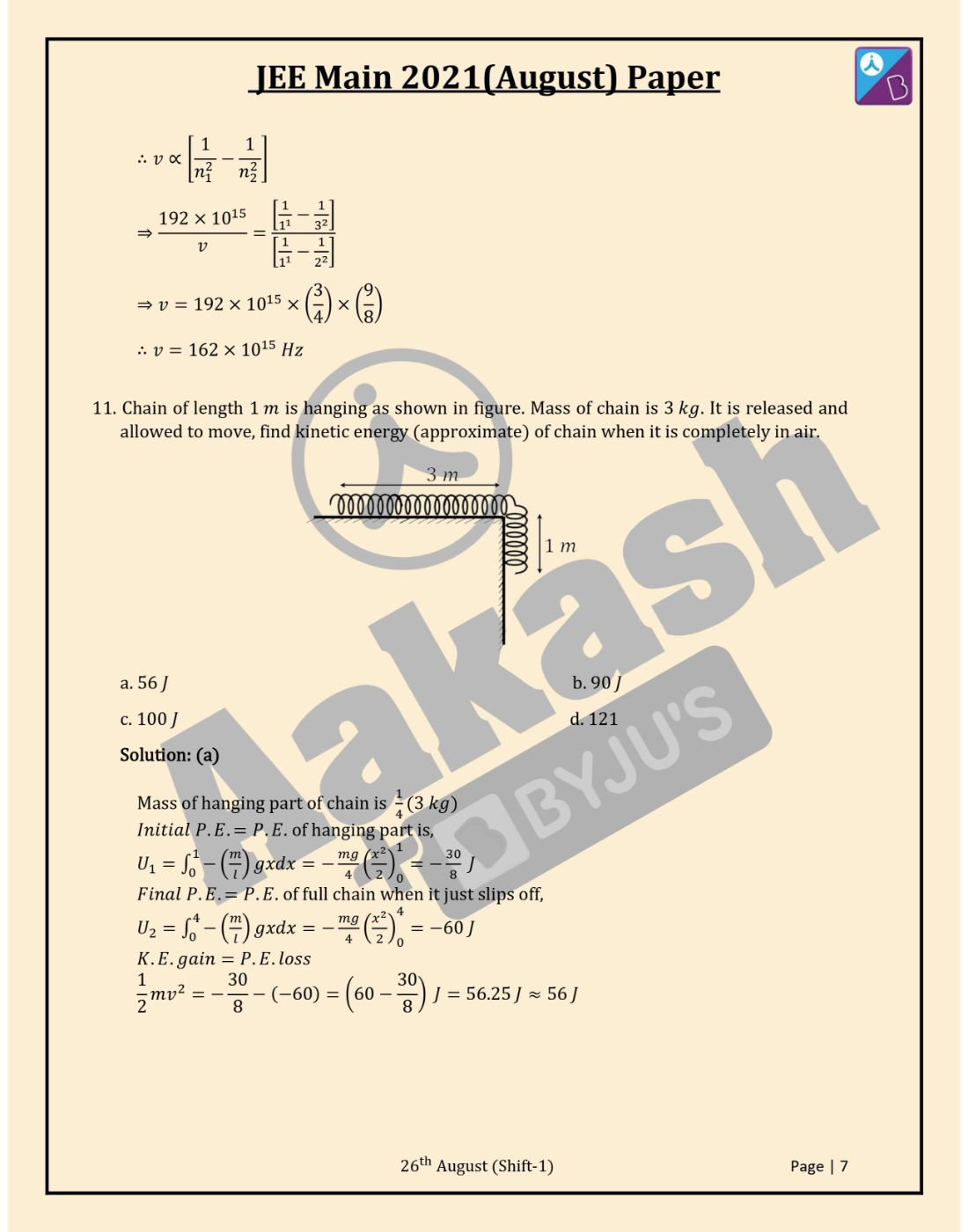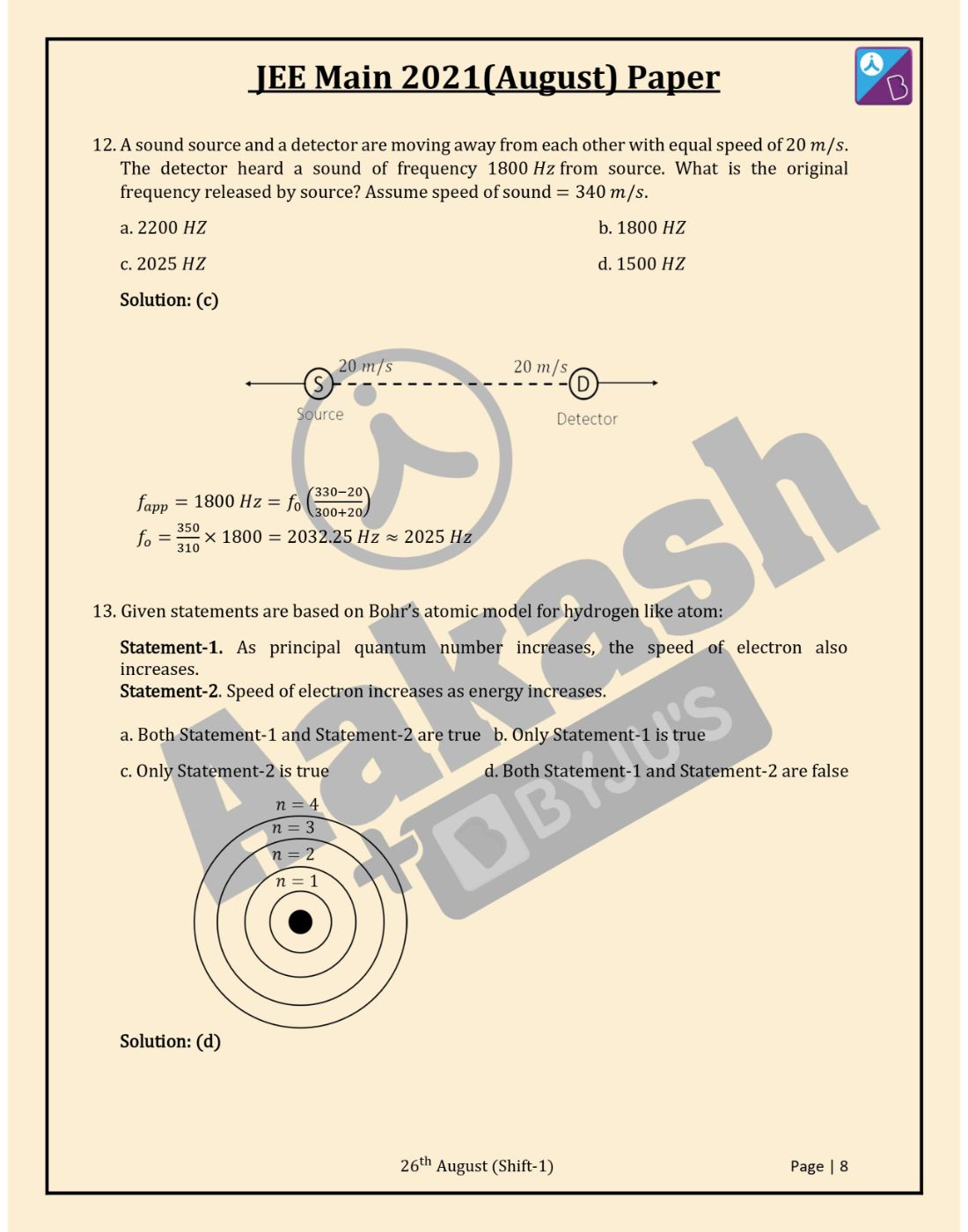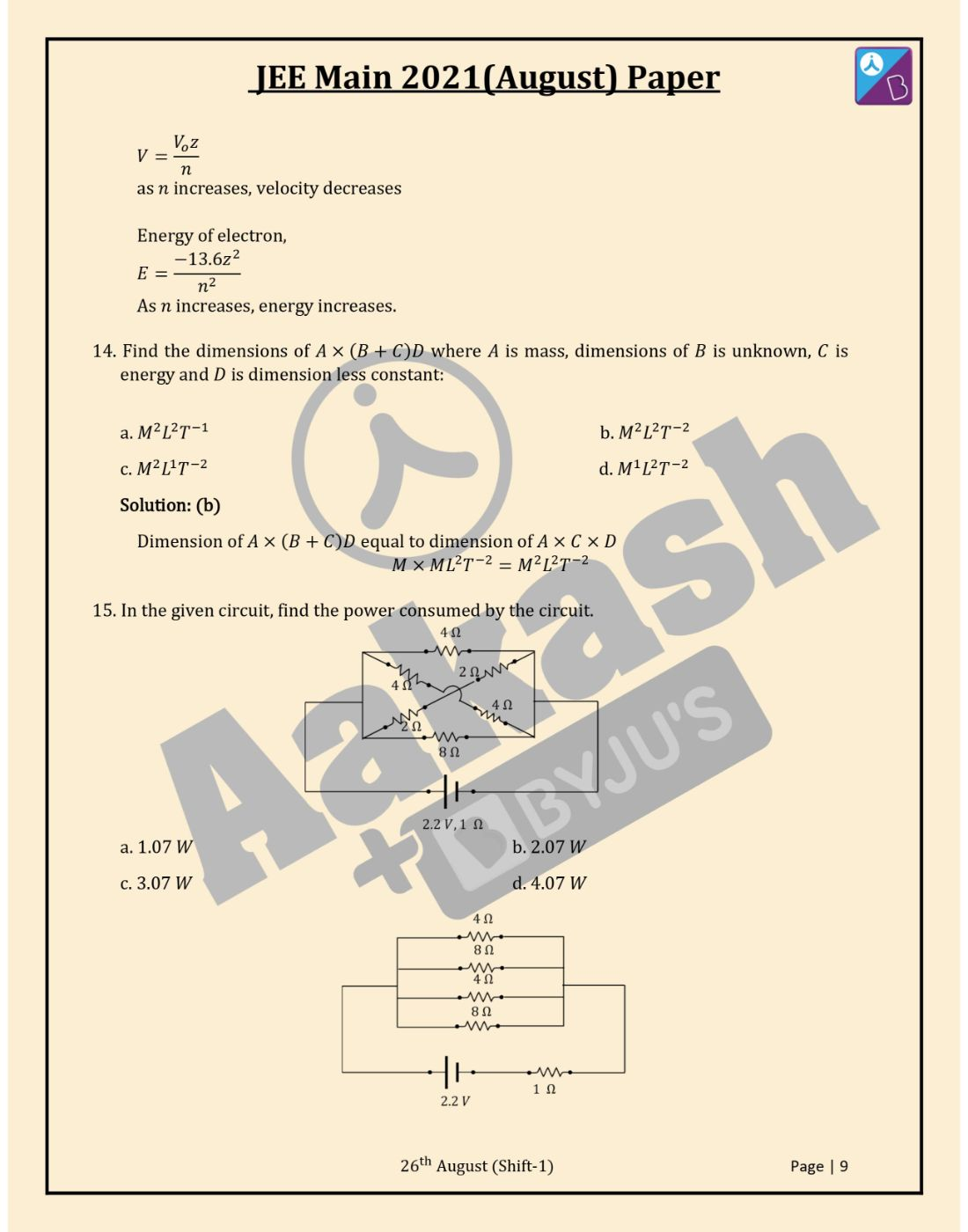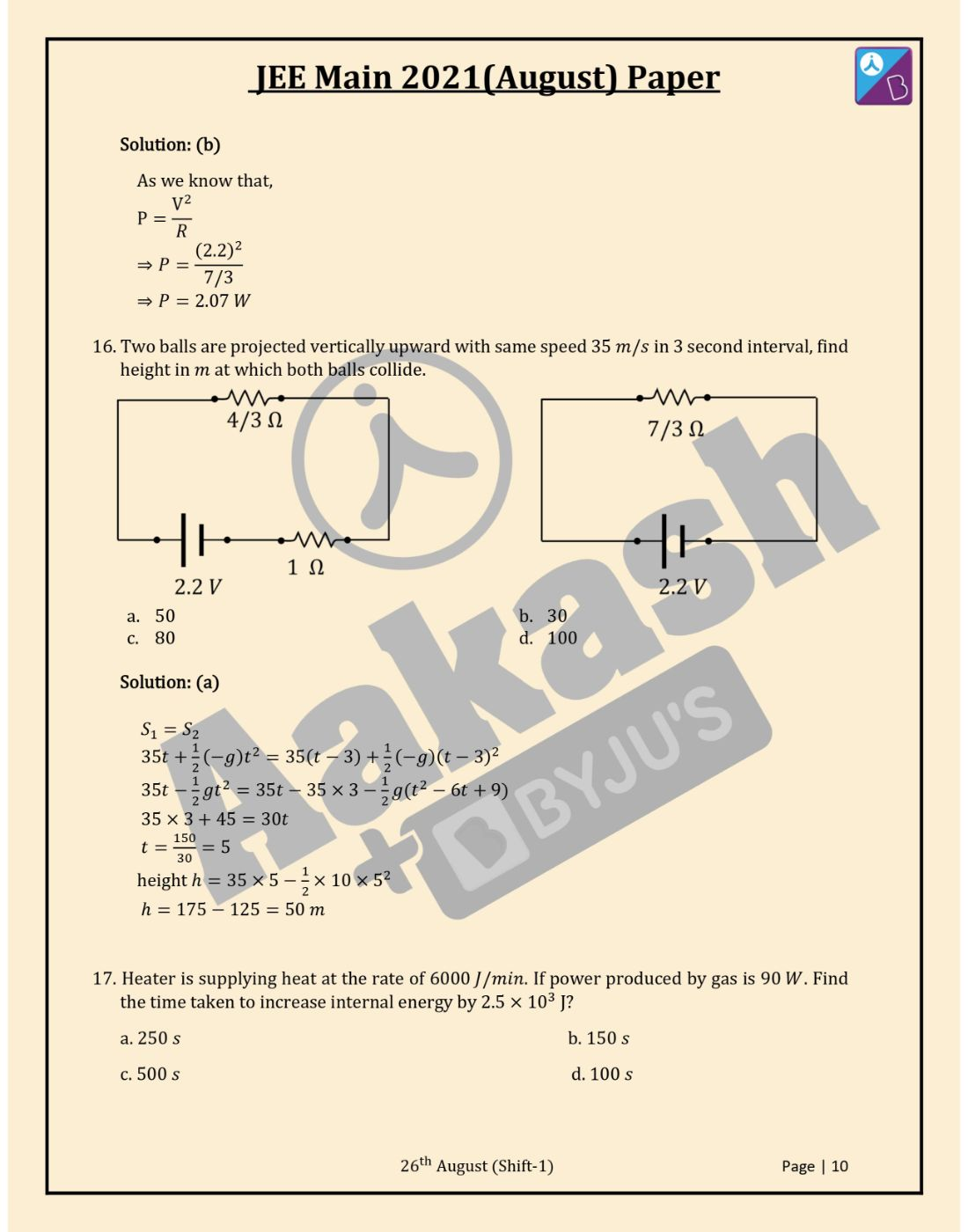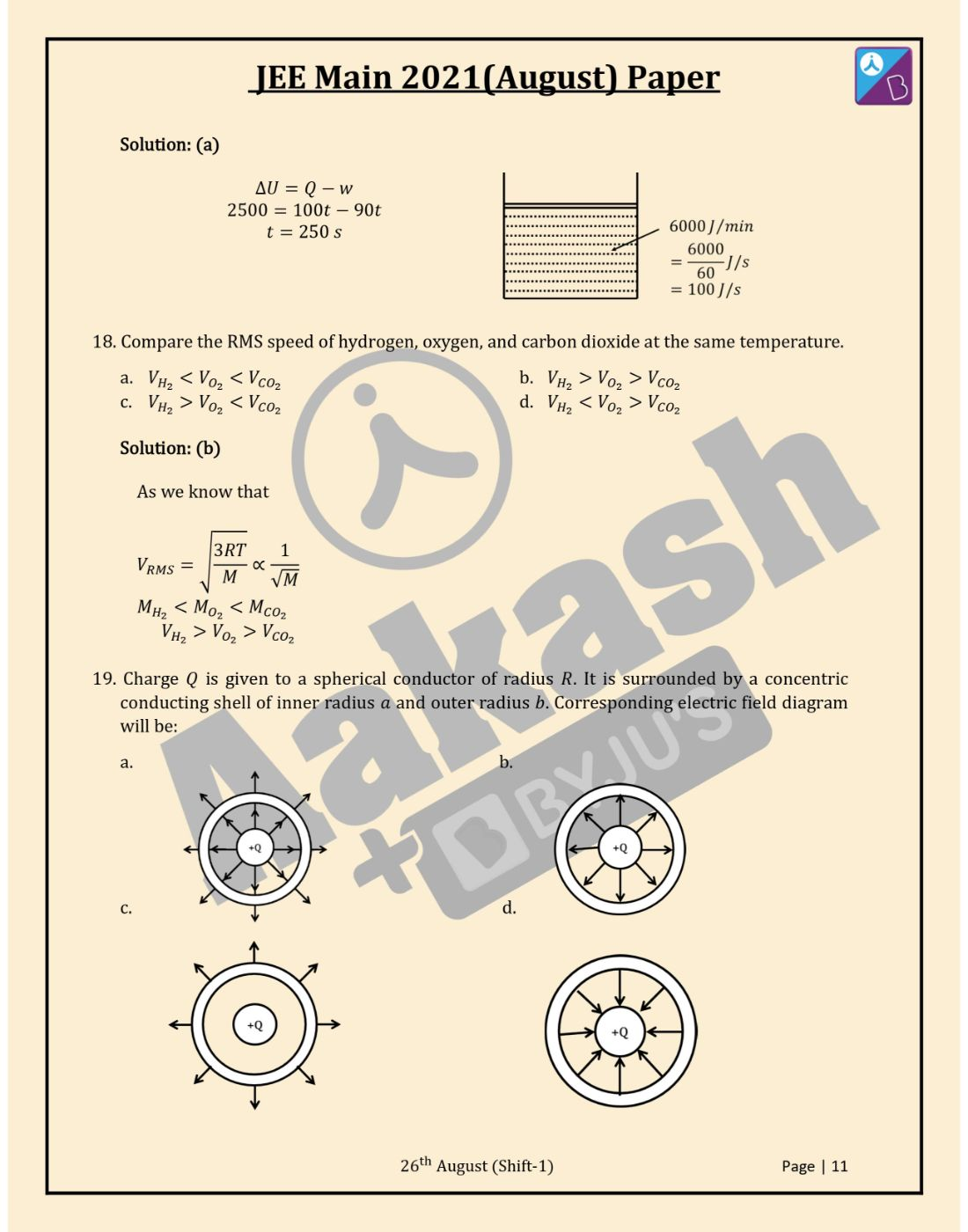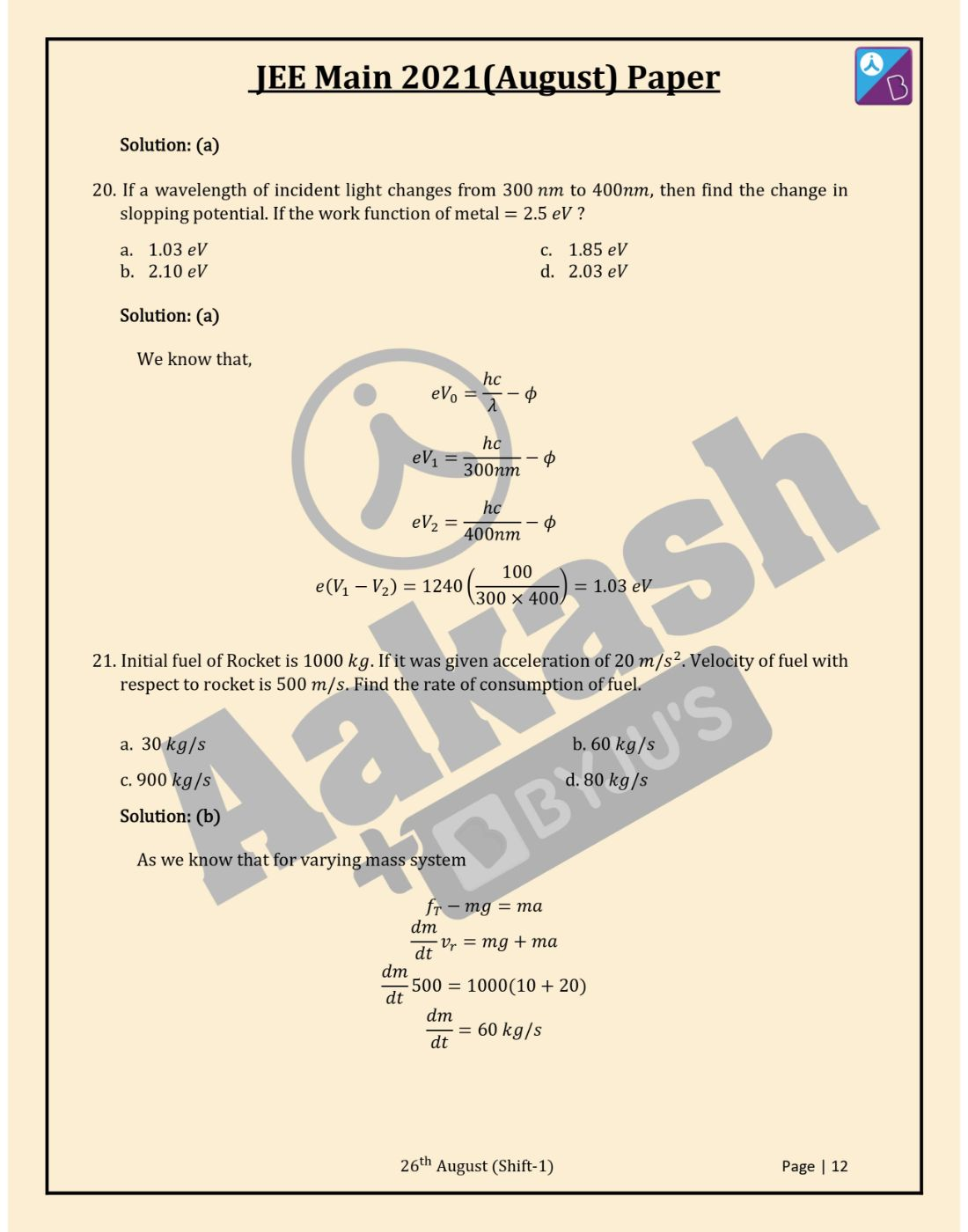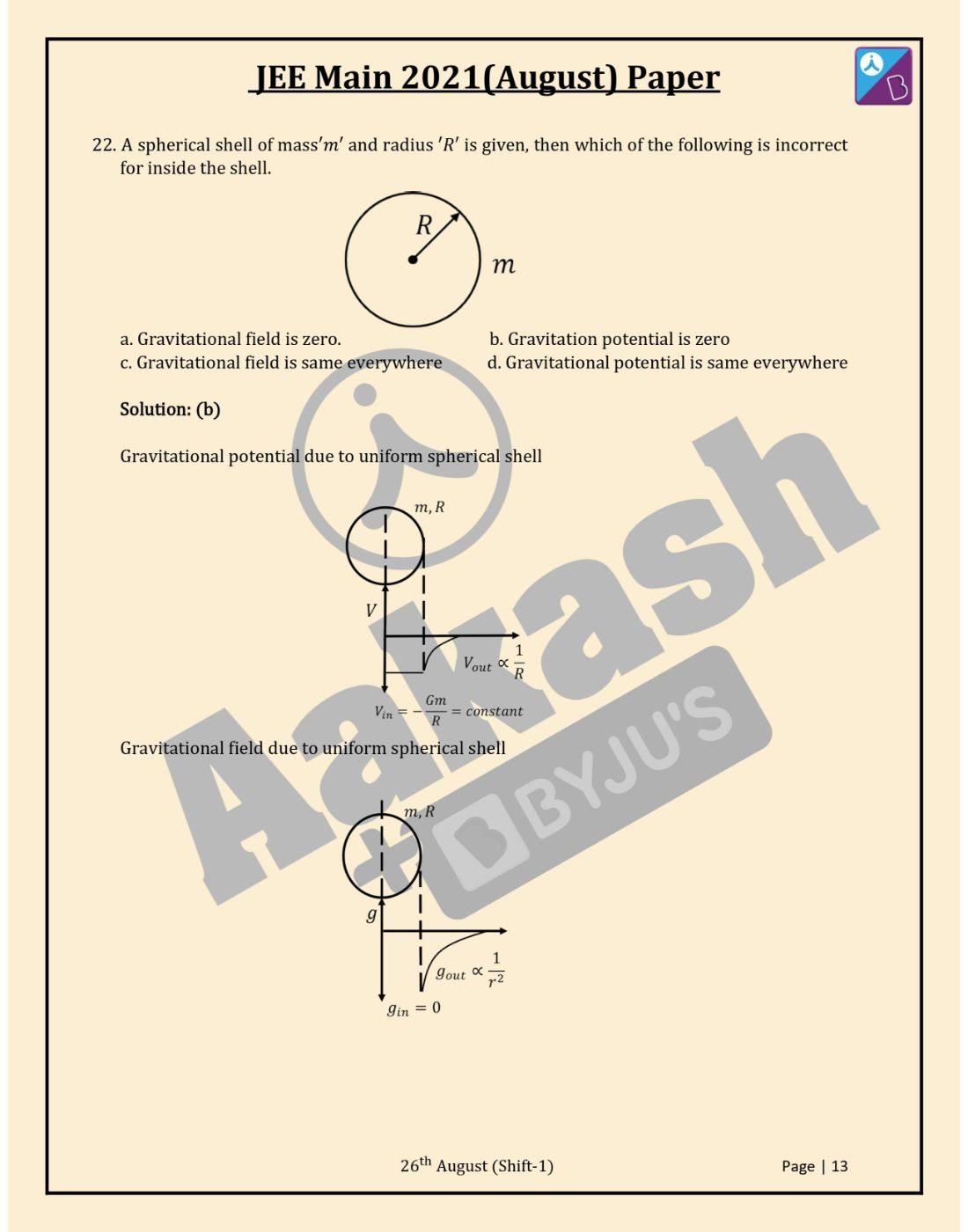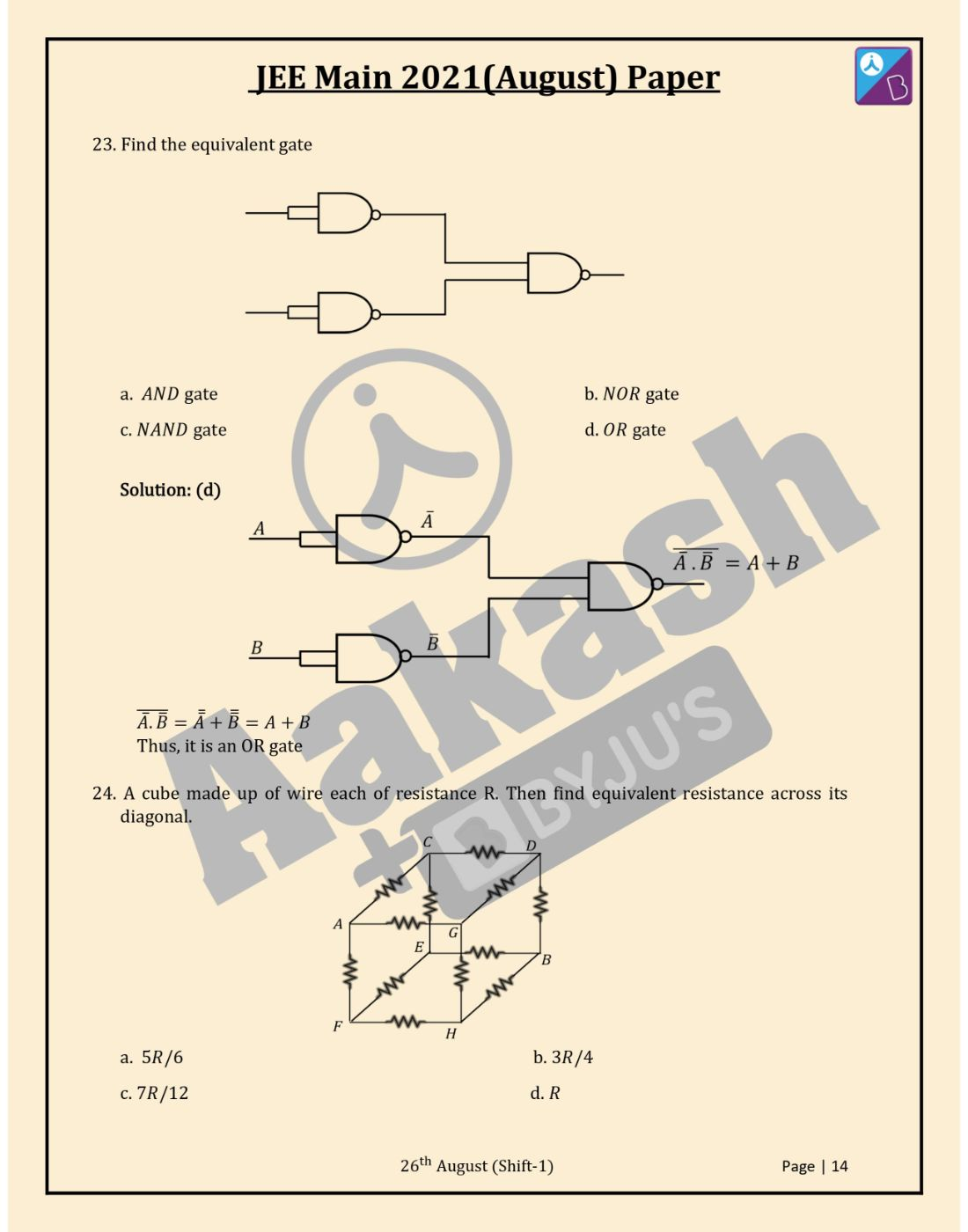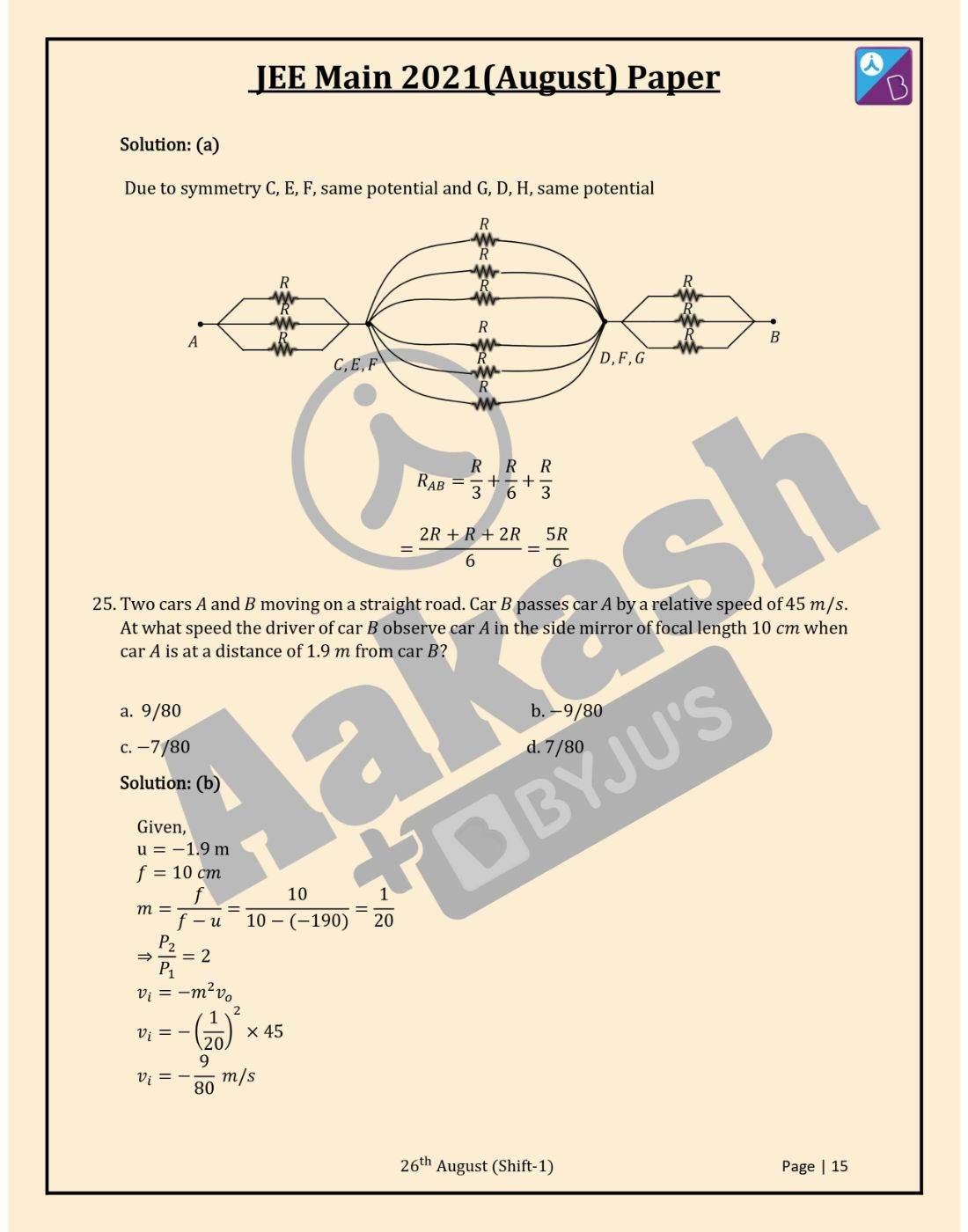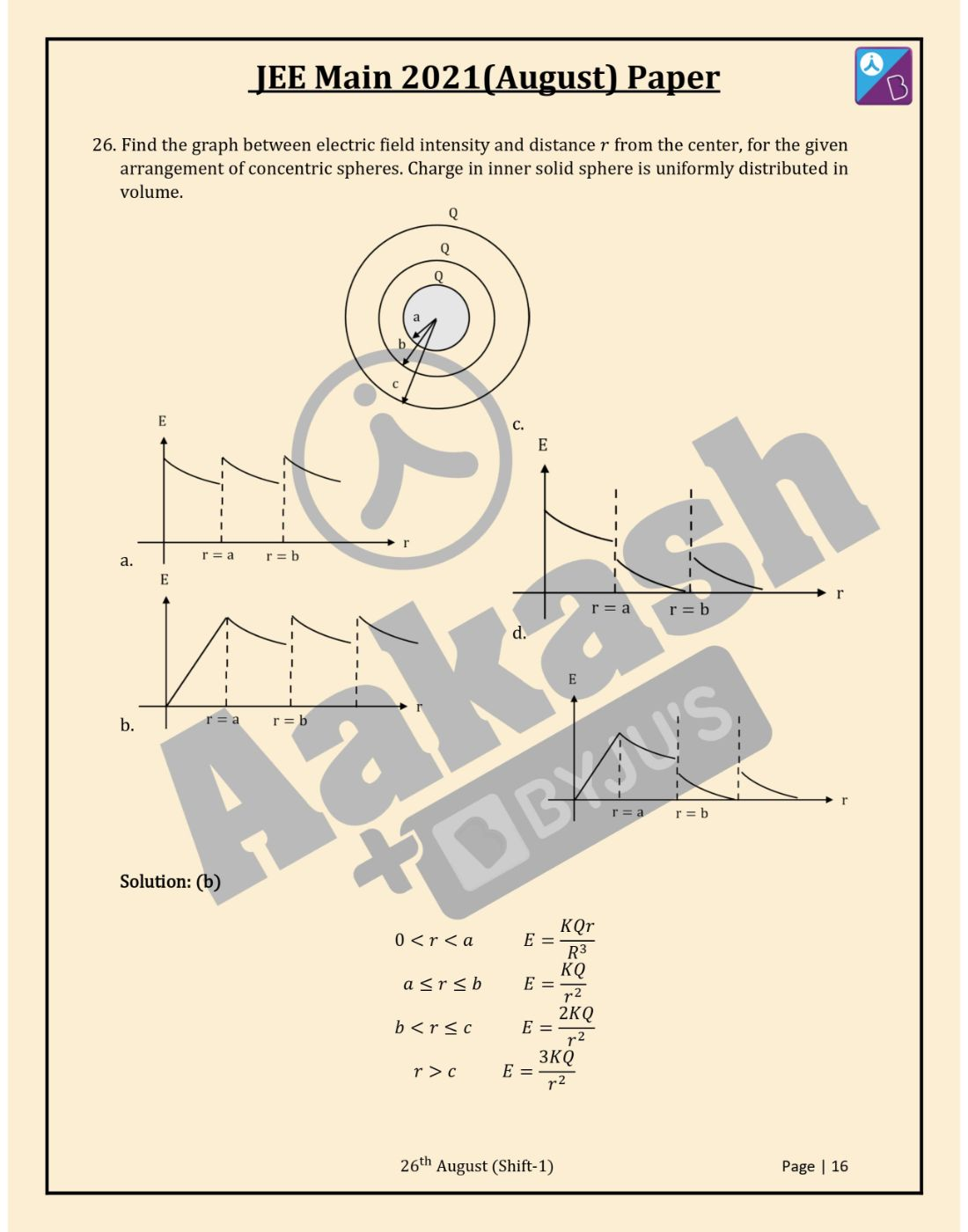Win up to 100% scholarship on Aakash BYJU'S JEE/NEET courses with ABNAT Win up to 100% scholarship on Aakash BYJU'S JEE/NEET courses with ABNAT

# JEE Main 2021 August 26 Shift 1 Physics Question Paper

JEE Main 2021 August 26th Shift 1 Physics Question Paper with Solutions is provided on this page. The candidates will find the memory-based questions of the physics paper here, they can refer to the answers and analyse their performance in the examination. All the answers are written in detail by the subject experts at BYJU’S. The PDF format of the solved question paper is also available for download. JEE aspirants can refer and practise these questions to score well in the examination.

JEE Main 2021 August 26th Shift 1 Physics Question Paper

Question 1: Find the moment of inertia for a badminton racket about an axis passing through ‘A’ and perpendicular to the plane as shown in the figure. Mass of the circular portion is M and that of the straight is;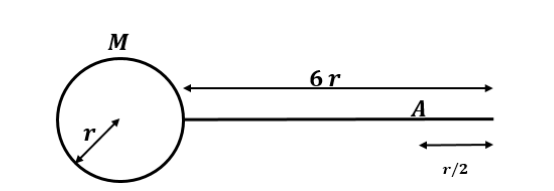a.

$$\begin{array}{l}\frac{173Mr^{2}}{4}+\frac{37mr^{2}}{4}\end{array}$$

b.
$$\begin{array}{l}-\frac{173Mr^{2}}{4}+\frac{37mr^{2}}{4}\end{array}$$

c.
$$\begin{array}{l}\frac{173mr^{2}}{4}+\frac{37Mr^{2}}{4}\end{array}$$

d.
$$\begin{array}{l}-\frac{173mr^{2}}{4}+\frac{37Mr^{2}}{4}\end{array}$$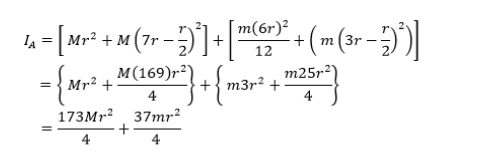Question 2: An amplitude modulated wave is represented by, Cm = 10(1 + 0.2sin12560t)sin(1.11 × 104t) V the modulating frequency in kHz will be;

As we know that,

$$\begin{array}{l}C_{m}= A_{c}\left [1+\frac{A_{m}}{A_{c}}sin2\pi f_{m}t \right ]\end{array}$$

⇒ 2πfm = 12560

fm = 2000 Hz = 2 kHz

Question 3: Two travelling waves produce a standing wave represented by equation y = 1.0mm cos(1.57cm−1 x) sin(78.5s−1) t, the node closest to the origin in the region x > 0 will be at x = _____cm.

At node y = 0

⇒ 1 cos(1.57 x) sin (178.5)t = 0

⇒ cos (1.57 x) = 0

⇒ 1.57 x = π/2

⇒ x = 1 cm

Question 4: Two wires of equal length with diameter 2 mm each having resistivity ρ1 = 12 μΩ. cm and ρ2 = 51 μΩ. cm respectively. They are connected in parallel so that their equivalent resistance is 3 Ω. Find the length of the wire.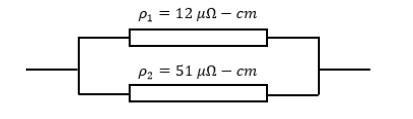a. 50 m
b. 97 m
c. 197 m
d. 291 m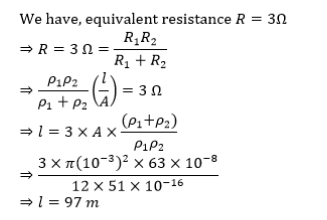Question 5: Assume an ideal gas is taken from the state from A to state B. The process is isothermal. Then the corresponding P-T graph will be;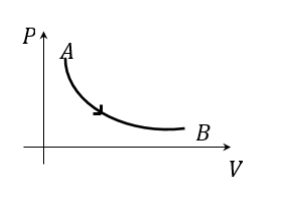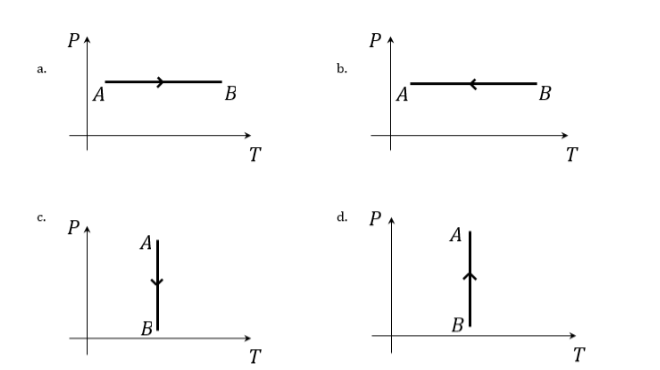As T = constant, so

P ∝ 1/V

Question 6:Find the difference in heights in limbs as shown in the given data. Surface tension T =7.3 × 10−2 N/m, the density of water ρ = 1000 Kg/m3 and the angle of contact θ = 0°.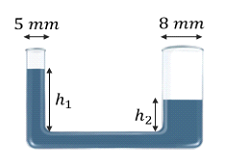a. 1.19 m
b. 2.19 m
c. 3.19 m
d. 4.19 m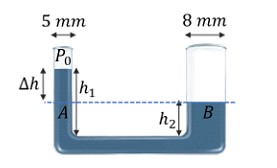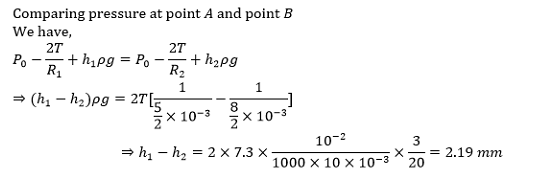Question 7: There is a soap bubble of radius 6 cm and it has another soap bubble of radius 3 cm inside it. Find the equivalent radius of the soap bubble which has the same excess pressure, as the pressure inside the bubble of radius 3 cm.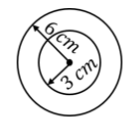a. 2 cm
b. 1 cm
c. 9 cm
d. 3 cm

We have from the given condition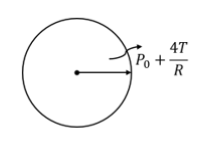$$\begin{array}{l}P_{0}+\frac{4T}{6}+\frac{4T}{3}=P_{0}+\frac{4T}{R}\end{array}$$

$$\begin{array}{l}\Rightarrow \frac{1}{6}+\frac{1}{3}=\frac{1}{R}\end{array}$$

⇒R = 2 cm

Question 8: In YDSE, d is the distance between slits and D is the distance between screen and slit plane. If y1 is the distance of 1st maxima from the central maxima for red light and y2 is the distance for violet light then what is the difference between the wavelength of red and violet?

a.

$$\begin{array}{l}\frac{(y_{1}+y_{2})d}{D}\end{array}$$

b.
$$\begin{array}{l}\frac{(y_{1}-y_{2})d}{D}\end{array}$$

c.
$$\begin{array}{l}\frac{(y_{1}-y_{2})D}{d}\end{array}$$

d.
$$\begin{array}{l}\frac{(y_{1}+y_{2})D}{d}\end{array}$$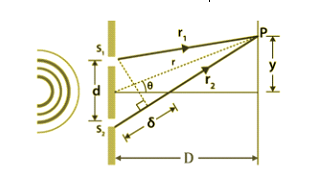From the condition of maximum we have

$$\begin{array}{l}y_{1}=\frac{\lambda _{r}D}{d}\end{array}$$
$$\begin{array}{l}y_{2}=\frac{\lambda _{v}D}{d}\end{array}$$

$$\begin{array}{l}\lambda _{r}-\lambda _{v}=\frac{(y_{1}-y_{2})d}{D}\end{array}$$

Question 9: Find the capacitance in a series LCR circuit in order to get maximum power. (XL = 250 Ω and f = 50 Hz).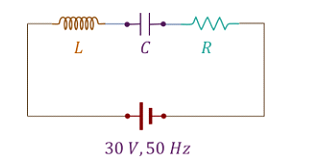a. 10.7 μF
b. 12.7 μF
c. 14.7 μF
d. 14.9 μF

As we know that, for maximum power, XC = XL (Condition of resonance)

⇒ 1/Cω = ωL

⇒ 1/Cω = 250

As, ω = 2πf = 2π x 50 = 100 π

⇒ C = 1/(100π x 250) = 12.7 μF

Question 10:For a hydrogen-like atom, the frequency of transition from n = 3 to n = 1 is 192 × 1015 Hz, then find the frequency of transition from n = 2 to n = 1

a. 162 x 1015 Hz
b. 226 x 1015 Hz
c. 162 x 1018 Hz
d. None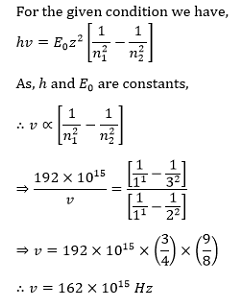Question 11: Chain of length 1 m is hanging as shown in the figure. The mass of the chain is 3 kg. It is released and allowed to move, find kinetic energy (approximate) of the chain when it is completely in the air.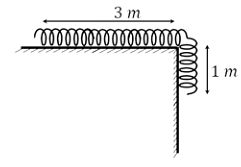a. 56 J
b. 90 J
c. 100 J
d. 121 J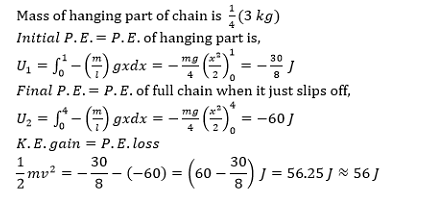Question 12: A sound source and a detector are moving away from each other with an equal speed of 20 m/s. The detector heard a sound of frequency 1800 Hz from the source. What is the original frequency released by the source? Assume speed of sound = 340 m/s.

a. 2200 HZ
b. 1800 HZ
c. 2025 HZ
d. 1500 HZ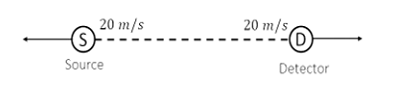fapp = 1800 Hz = f0 ((330−20)/(300+20))

fo = (350/310)× 1800 = 2032.25 Hz ≈ 2025 Hz

Question 13: Given statements are based on Bohr’s atomic model for hydrogen-like atoms:

Statement-1. As the principal quantum number increases, the speed of the electron also

increases.

Statement-2. Speed of electron increases as energy increases.

a. Both Statement-1 and Statement-2 are true
b. Only Statement-1 is true
c. Only Statement-2 is true
d. Both Statement-1 and Statement-2 are false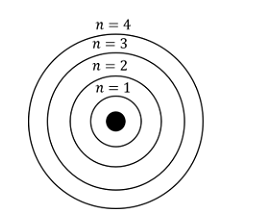V = V0z/n

as n increases, velocity increases

The energy of electrons,

$$\begin{array}{l}E = \frac{-13.6z^{2}}{n^{2}}\end{array}$$

As n increases, energy increases

Question 14: Find the dimensions of A × (B + C)D where A is mass, dimensions of B is unknown, C is energy and D is dimensionless constant:

a. M2L2T−1
b. M2L2T−2
c. M2L1T−2
d. M1L2T−2

Dimension of A × (B + C)D equal to dimension of A × C × D

M × ML2T−2 = M2L2T−2

Question 15: In the given circuit, find the power consumed by the circuit.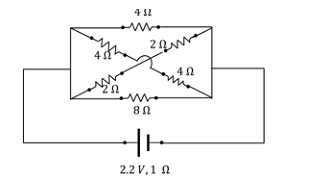a. 1.07 W
b. 2.07 W
c. 3.07 W
d. 4.07 W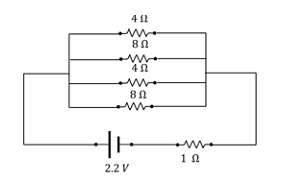As we know that,

P = V2/R

⇒ P = (2.2)2/(7/3)

⇒ P = 2.07 W

Question 16:Two balls are projected vertically upward with the same speed 35 m/s in a 3-second interval, find height in m at which both balls collide.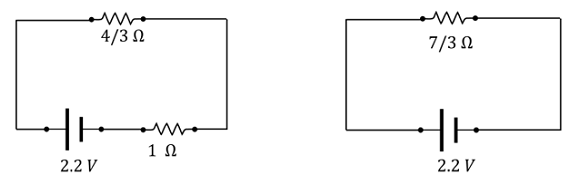a. 50
b. 30
c. 80
d. 100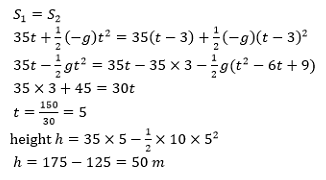Question 17: A heater is supplying heat at the rate of 6000 J/min. If power produced by gas is 90 W. Find the time taken to increase internal energy by 2.5 × 103 J?

a. 250 s
b. 150 s
c. 500 s
d. 100 s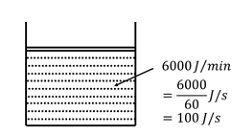?U = Q – w

2500 = 100t – 90t

t = 250 s

Question 18: Compare the RMS speed of hydrogen, oxygen, and carbon dioxide at the same temperature.

a. VH2 < VO2 < VCO2
b. VH2 > VO2 > VCO2
c. VH2 > VO2 < VCO2
d. VH2 < VO2 > VCO2

As we know that

$$\begin{array}{l}V_{RMS}=\sqrt{\frac{3RT}{M}}\end{array}$$
∝ 1/√M

MH2 < MO2 < MCO2

VH2 > VO2 > VCO2

Question 19: Charge Q is given to a spherical conductor of radius R. It is surrounded by a concentric conducting shell of inner radius a and outer radius b. Corresponding electric field diagram will be: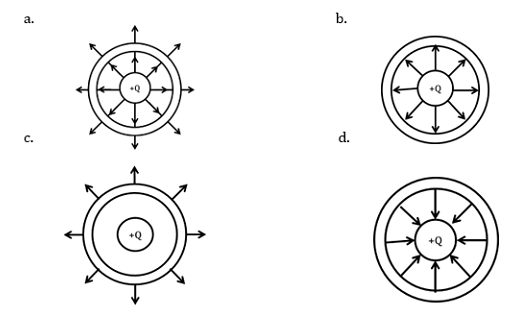Question 20: If a wavelength of incident light changes from 300 nm to 400nm, then find the change in sloping potential. If the work function of metal = 2.5 eV?

a. 1.03 eV
b. 2.10 eV
c. 1.85 eV
d. 2.03 eV

We know that,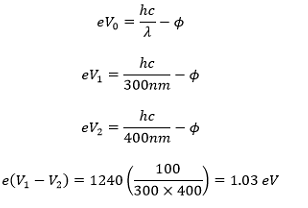Question 21: Initial fuel in a rocket is 1000 kg. If it was given an acceleration of 20 m/s2 the velocity of fuel with respect to the rocket is 500 m/s. Find the rate of consumption of fuel.

a. 30 kg/s
b. 60 kg/s
c. 900 kg/s
d. 80 kg/s

As we know that for varying mass system

fT − mg = ma

(dm/dt) vr = mg + ma

(dm/dt) 500 = 1000(10 + 20)

(dm/dt) = 60 kg/s

Question 22: A spherical shell of mass′m′ and radius ′R′ is given, then which of the following is incorrect for inside the shell?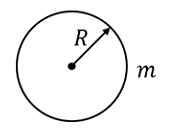a. Gravitational field is zero
b. Gravitation potential is zero
c. The gravitational field is the same everywhere
d. Gravitational potential is the same everywhere

Gravitational potential due to a uniform spherical shell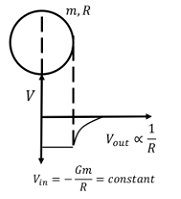Gravitational field due to a uniform spherical shell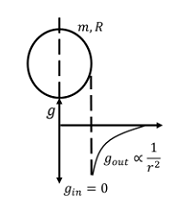Question 23: Find the equivalent gate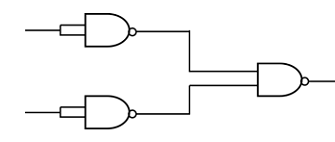a. AND gate
b. NOR gate
c. NAND gate
d. OR gate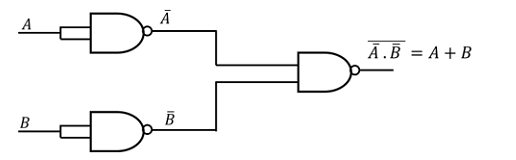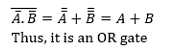Question 24: A cube made up of wire each of resistance R. Then find equivalent resistance across its diagonal.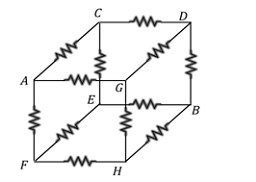a. 5R/6
b. 3R/4
c. 7R/12
d. R

Due to symmetry C, E, F, same potential and G, D, H, same potential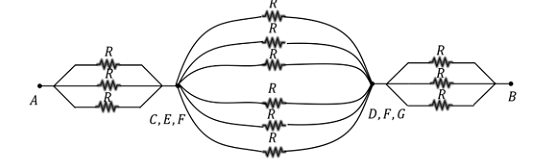$$\begin{array}{l}R_{AB}=\frac{R}{3}+\frac{R}{6}+\frac{R}{3}\end{array}$$
$$\begin{array}{l}R_{AB}=\frac{5R}{6}\end{array}$$

Question 25: Two cars A and B moving on a straight road. Car B passes car A by a relative speed of 45 m/s. At what speed does the driver of car B observe car A in the side mirror of focal length 10 cm when car A is at a distance of 1.9 m from car B?

a. 9/80
b. −9/80
c. −7/80
d. 7/80

Given,

u = −1.9 m

f = 10 cm

m = f/(f − u)

=10/(10 − (−190))

=1/20

⇒P2/P1 = 2

vi = −m2vo

vi = − (1/20)2× 45

vi = −9/80 m/s

Question 26:Find the graph between electric field intensity and distance r from the centre, for the given arrangement of concentric spheres. Charge in the inner solid sphere is uniformly distributed in volume.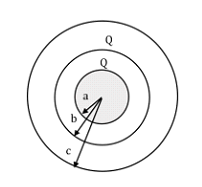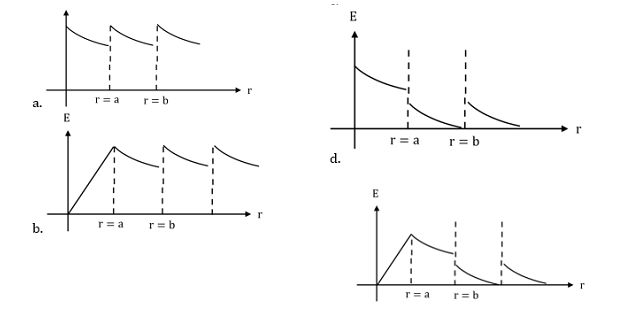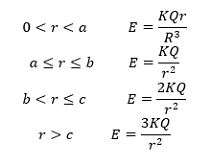#### Video Solutions – JEE Main 2021 Question Papers